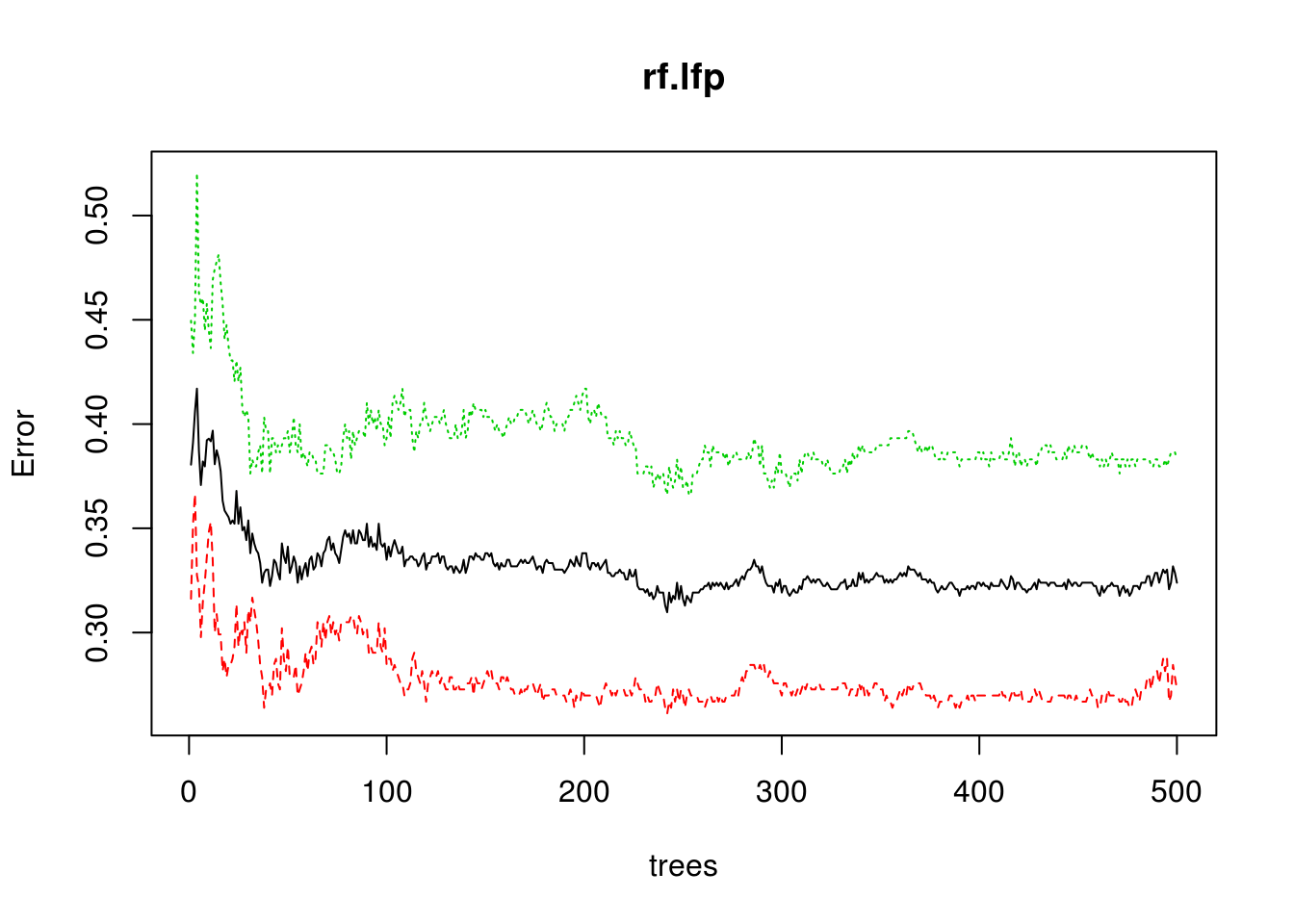# Random Forest Classification in R

This post will cover the use of random forest for classification. Random forest involves the use of many decision trees in the development of a classification or regression tree. The results of each individual tree are added together and the mean is used in the final classification of an example. The use of an ensemble helps in dealing with the bias-variance tradeoff.In the example of random forest classification, we will use the “Participation” dataset from the “ecdat” package. We want to classify people by their labor participation based on the other variables available in the dataset. Below is some initial code

``library(randomForest);library(Ecdat)``
``````data("Participation")
str(Participation)``````
``````## 'data.frame':    872 obs. of  7 variables:
##  \$ lfp    : Factor w/ 2 levels "no","yes": 1 2 1 1 1 2 1 2 1 1 ...
##  \$ lnnlinc: num  10.8 10.5 11 11.1 11.1 ...
##  \$ age    : num  3 4.5 4.6 3.1 4.4 4.2 5.1 3.2 3.9 4.3 ...
##  \$ educ   : num  8 8 9 11 12 12 8 8 12 11 ...
##  \$ nyc    : num  1 0 0 2 0 0 0 0 0 0 ...
##  \$ noc    : num  1 1 0 0 2 1 0 2 0 2 ...
##  \$ foreign: Factor w/ 2 levels "no","yes": 1 1 1 1 1 1 1 1 1 1 ...``````

For the data preparation, we need to multiple age by ten as the current values imply small children. Furthermore, we need to change the “lnnlinc” variable from the log of salary to just the regular salary. After completing these two steps, we need to split our data into training and testing sets. Below is the code

``````Participation\$age<-10*Participation\$age #normal age
Participation\$lnnlinc<-exp(Participation\$lnnlinc) #actual income not log
#split data
set.seed(502)
ind=sample(2,nrow(Participation),replace=T,prob=c(.7,.3))
train<-Participation[ind==1,]
test<-Participation[ind==2,]``````

We will now create our classification model using random forest.

``````set.seed(123)
rf.lfp<-randomForest(lfp~.,data = train)
rf.lfp``````
``````##
## Call:
##  randomForest(formula = lfp ~ ., data = train)
##                Type of random forest: classification
##                      Number of trees: 500
## No. of variables tried at each split: 2
##
##         OOB estimate of  error rate: 32.39%
## Confusion matrix:
##      no yes class.error
## no  248  93   0.2727273
## yes 113 182   0.3830508``````

The output is mostly self-explanatory. It includes the number of trees, number of variables at each split, error rate, and the confusion matrix. In general, are error rate is poor and we are having a hard time distinguishing between those who work and do not work based on the variables in the dataset. However, this is based on having all 500 trees in the analysis. Having this many trees is probably not necessary but we need to confirm this.

We can also plot the error by tree using the “plot” function as shown below.

``plot(rf.lfp)``It looks as though error lowest with around 400 trees. We can confirm this using the “which.min” function and call information from “err.rate” in our model.

``which.min(rf.lfp\$err.rate[,1])``
``##  242``

We need 395 trees in order to reduce the error rate to its most optimal level. We will now create a new model that contains 395 trees in it.

``````rf.lfp2<-randomForest(lfp~.,data = train,ntree=395)
rf.lfp2``````
``````##
## Call:
##  randomForest(formula = lfp ~ ., data = train, ntree = 395)
##                Type of random forest: classification
##                      Number of trees: 395
## No. of variables tried at each split: 2
##
##         OOB estimate of  error rate: 31.92%
## Confusion matrix:
##      no yes class.error
## no  252  89   0.2609971
## yes 114 181   0.3864407``````

The results are mostly the same. There is a small decline in error but not much to get excited about. We will now run our model on the test set.

``````rf.lfptest<-predict(rf.lfp2,newdata=test,type = 'response')
table(rf.lfptest,test\$lfp)``````
``````##
## rf.lfptest no yes
##        no  93  48
##        yes 37  58``````
``(92+63)/(92+63+43+38) #calculate accuracy``
``##  0.6567797``

Still disappointing, there is one last chart we should examine and that is the importance of each variable plot. It shows which variables are most useful in the prediction process. Below is the code.

``varImpPlot(rf.lfp2)``This plot clearly indicates that salary (“lnnlinc”), age, and education are the strongest features for classifying by labor activity. However, the overall model is probably not useful.

Conclusion

This post explained and demonstrated how to conduct a random forest analysis. This form of analysis is powerful in dealing with large datasets with nonlinear relationships among the variables.

## 1 thought on “Random Forest Classification in R”

1.auzout

Reblogged this on Applied Behavioral and Moral Thought and commented:
Very interesting…!